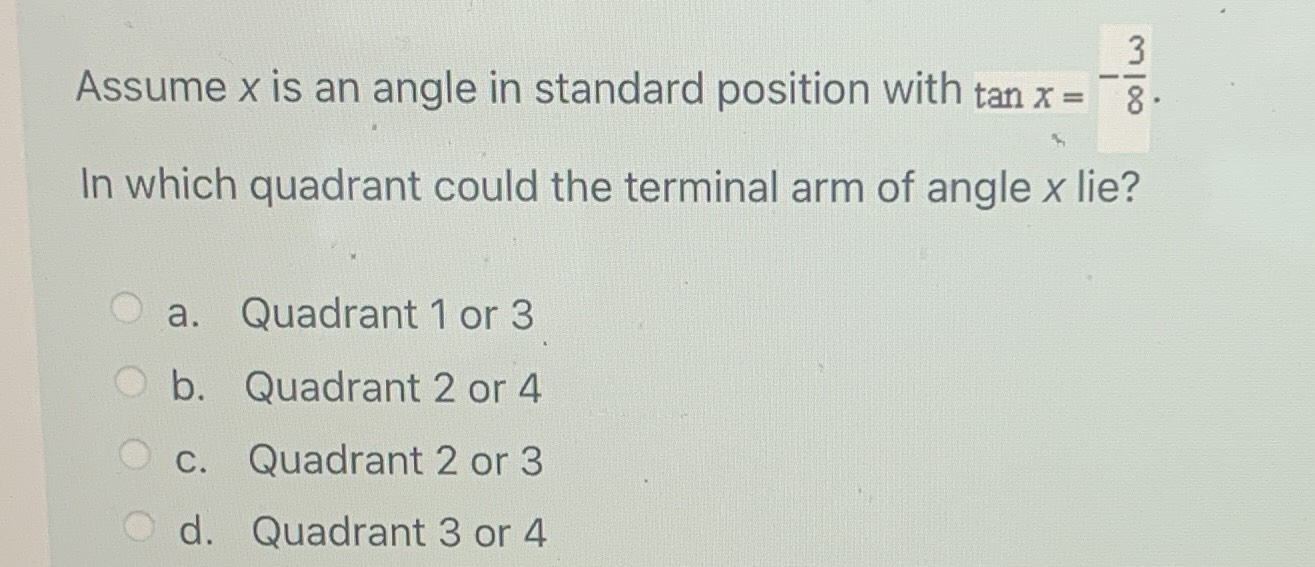### Still have math questions?

Trigonometry
QuestionAssume $$x$$ is an angle in standard position with $$\tan x = - \frac { 3 } { 8 }$$

In which quadrant could the terminal arm of angle $$x$$ lie?

a. Quadrant $$1$$ or $$3$$

b. Quadrant $$2$$ or $$4$$

c. Quadrant $$2$$ or $$3$$

d. Quadrant $$3$$ or $$4$$